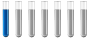## How to add attachment, use LaTeX, write chemical equations in this forum?

Chemistry and homework help forum.

Organic Chemistry, Analytical Chemistry, Biochemistry, Physical Chemistry, Computational Chemistry, Theoretical Chemistry, High School Chemistry, Colledge Chemistry and University Chemistry Forum.

Share your chemistry ideas, discuss chemical problems, ask for help with scientific chemistry questions, inspire others by your chemistry vision!

Please feel free to start a scientific chemistry discussion here!

Discuss chemistry homework problems with experts!

Ask for help with chemical questions and help others with your chemistry knowledge!

Moderators: expert, ChenBeier, Xen

Dhamnekar Winod
NewbiePosts: 1
Joined: Sat Nov 21, 2020 10:14 am

### How to add attachment, use LaTeX, write chemical equations in this forum?

How to add attachment, use LaTeX and write chemical equations in this forum?
ChenBeier
Distinguished MemberPosts: 460
Joined: Wed Sep 27, 2017 7:25 am
Location: Berlin, Germany

### Re: How to add attachment, use LaTeX, write chemical equations in this forum?

Impossible.
You can attach img, or URL. Icon on top.
Posts: 11
Joined: Sat May 13, 2006 8:47 am
Contact:

### Re: How to add attachment, use LaTeX, write chemical equations in this forum?

Hi Dhamnekar,

Do you mind clarifying your use case, what is a specific example of what you would like to write?

One can write chemical equation directly in post body, e.g.:
2 KMnO4 + 16 HCl = 2 KCl + 2 MnCl2 + 8 H2O + 5 Cl2
ChenBeier
Distinguished MemberPosts: 460
Joined: Wed Sep 27, 2017 7:25 am
Location: Berlin, Germany

### Re: How to add attachment, use LaTeX, write chemical equations in this forum?

How to get Ion like S 2-. The 2- in exponent.
Attachment of PDF is it possible?
Posts: 11
Joined: Sat May 13, 2006 8:47 am
Contact:

### Re: How to add attachment, use LaTeX, write chemical equations in this forum?

We enabled attachemeneta and added support latex, formula and equation:

Code: Select all

[formula]H2SO4[/formula]
H2SO4 📖

Code: Select all

[equation] 2 KMnO4 + 16 HCl = 2 KCl + 2 MnCl2 + 8 H2O + 5 Cl2[/equation]
2 KMnO4 + 16 HCl = 2 KCl + 2 MnCl2 + 8 H2O + 5 Cl2 📖

Code: Select all

$\frac{\partial f}{\partial x} = 2\,\sqrt{a}\,x$

$$\frac{\partial f}{\partial x} = 2\,\sqrt{a}\,x$$
ChenBeier
Distinguished MemberPosts: 460
Joined: Wed Sep 27, 2017 7:25 am
Location: Berlin, Germany

### Re: How to add attachment, use LaTeX, write chemical equations in this forum?

Great, but it needs some explanation of the syntax how to do.
Posts: 11
Joined: Sat May 13, 2006 8:47 am
Contact:

### Re: How to add attachment, use LaTeX, write chemical equations in this forum?

Latex math syntax:
https://en.wikibooks.org/wiki/LaTeX/Mathematics

Ions are also supported now, example:

Code: Select all

[equation]Ca{2+} + SO4{2-} = CaSO4[/equation]
Ca2+ + SO42- = CaSO4 📖
ChenBeier
Distinguished MemberPosts: 460
Joined: Wed Sep 27, 2017 7:25 am
Location: Berlin, Germany

### Re: How to add attachment, use LaTeX, write chemical equations in this forum?

Great Sounds Good.